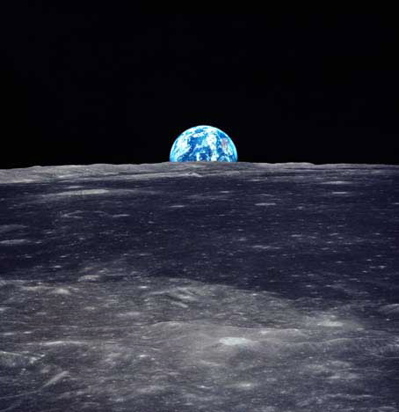## Chapter 4. Global image features

The task in this tutorial is to understand how we can extract numerical representations from images and how these numerical representations can be used to provide similarity measures between images, so that we can, for example, find the most similar images from a set.

As you know, images are made up of pixels which are basically numbers that represent a colour. This is the most basic form of numerical representation of an image. However, we can do calculations on the pixel values to get other numerical representations that mean different things. In general, these numerical representations are known as feature vectors and they represent particular features.

Let’s take a very common and easily understood type of feature. It’s called a colour histogram and it basically tells you the proportion of different colours within an image (e.g. 90% red, 5% green, 3% orange, and 2% blue). As pixels are represented by different amounts of red, green and blue we can take these values and accumulate them in our histogram (e.g. when we see a red pixel we add 1 to our red pixel count in the histogram).

A histogram can accrue counts for any number of colours in any number of dimensions but the usual is to split the red, green and blue values of a pixel into a smallish number of bins into which the colours are thrown. This gives us a three-dimensional cube, where each small cubic bin is accruing counts for that colour.

OpenIMAJ contains a multidimensional `MultidimensionalHistogram` implementation that is constructed using the number of bins required in each dimension. For example:

`MultidimensionalHistogram histogram = new MultidimensionalHistogram( 4, 4, 4 );`

This code creates a histogram that has 64 (4 × 4 × 4) bins. However, this data structure does not do anything on its own. The `HistogramModel` class provides a means for creating a `MultidimensionalHistogram` from an image. The `HistogramModel` class assumes the image has been normalised and returns a normalised histogram:

```HistogramModel model = new HistogramModel( 4, 4, 4 );
model.estimateModel( image );
MultidimensionalHistogram histogram = model.histogram;```

You can print out the histogram to see what sort of numbers you get for different images. Note that the you can re-use the `HistogramModel` by applying it to different images. If you do reuse the `HistogramModel` the `model.histogram` will be the same object, so you'll need to `clone()` it if you need to keep hold of its values for multiple images. Let’s load in 3 images then generate and store the histograms for them:

```URL[] imageURLs = new URL[] {
new URL( "http://openimaj.org/tutorial/figs/hist1.jpg" ),
new URL( "http://openimaj.org/tutorial/figs/hist2.jpg" ),
new URL( "http://openimaj.org/tutorial/figs/hist3.jpg" )
};

List<MultidimensionalHistogram> histograms = new ArrayList<MultidimensionalHistogram>();
HistogramModel model = new HistogramModel(4, 4, 4);

for( URL u : imageURLs ) {
}```We now have a list of histograms from our images. The `Histogram` class extends a class called the `MultidimensionalDoubleFV` which is a feature vector represented by multidimensional set of double precision numbers. This class provides us with a `compare()` method which allows comparison between two multidimensional sets of doubles. This method takes the other feature vector to compare against and a comparison method which is implemented in the `DoubleFVComparison` class.

So, we can compare two histograms using the Euclidean distance measure like so:

`double distanceScore = histogram1.compare( histogram2, DoubleFVComparison.EUCLIDEAN );`

This will give us a score of how similar (or dissimilar) the histograms are. It’s useful to think of the output score as a distance apart in space. Two very similar histograms will be very close together so have a small distance score, whereas two dissimilar histograms will be far apart and so have a large distance score.

The Euclidean distance measure is symmetric (that is, if you compare `histogram1` to `histogram2` you will get the same score if you compare `histogram2` to `histogram1`) so we can compare all the histograms with each other in a simple, efficient, nested loop:

```for( int i = 0; i < histograms.size(); i++ ) {
for( int j = i; j < histograms.size(); j++ ) {
double distance = histograms.get(i).compare( histograms.get(j), DoubleFVComparison.EUCLIDEAN );
}
}```

## 4.1. Exercises

### 4.1.1. Exercise 1: Finding and displaying similar images

Which images are most similar? Does that match with what you expect if you look at the images? Can you make the application display the two most similar images that are not the same?

### 4.1.2. Exercise 2: Exploring comparison measures

What happens when you use a different comparison measure (such as `DoubleFVComparison.INTERSECTION`)?